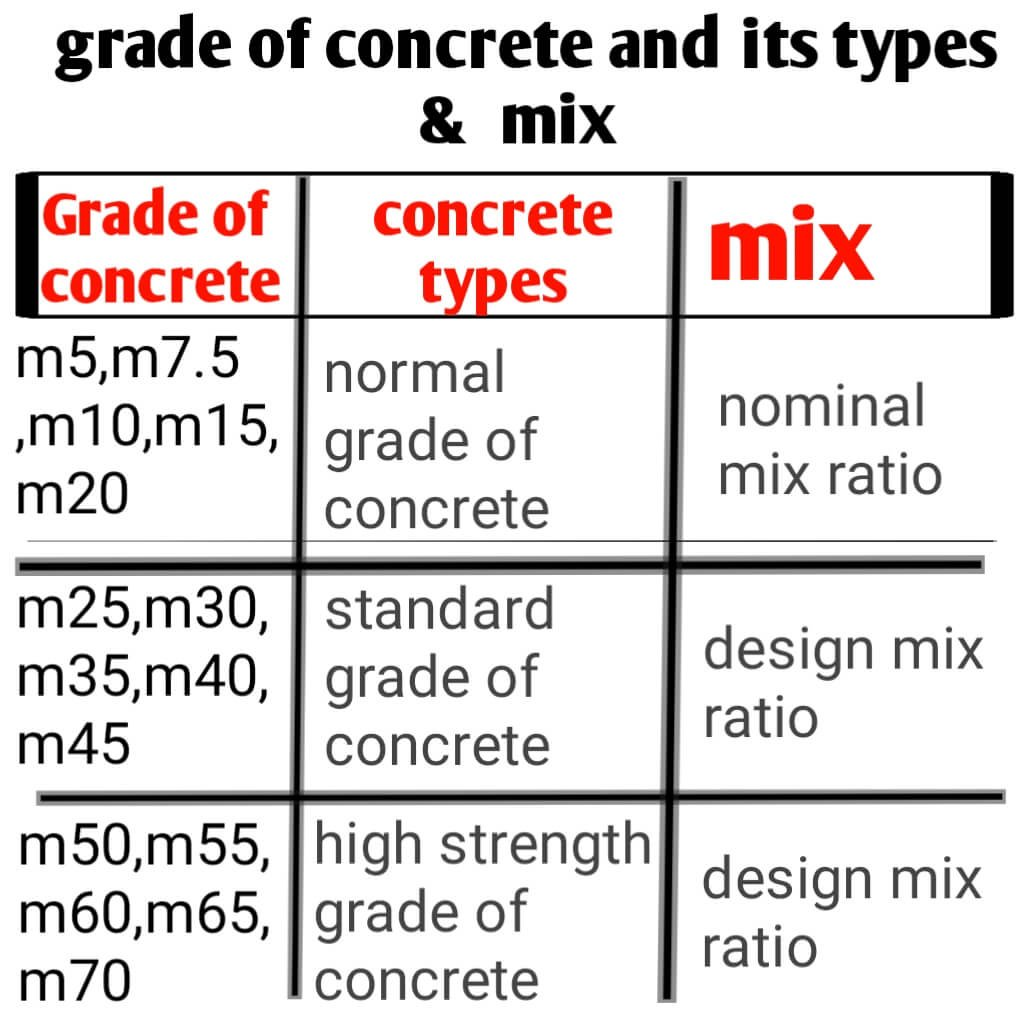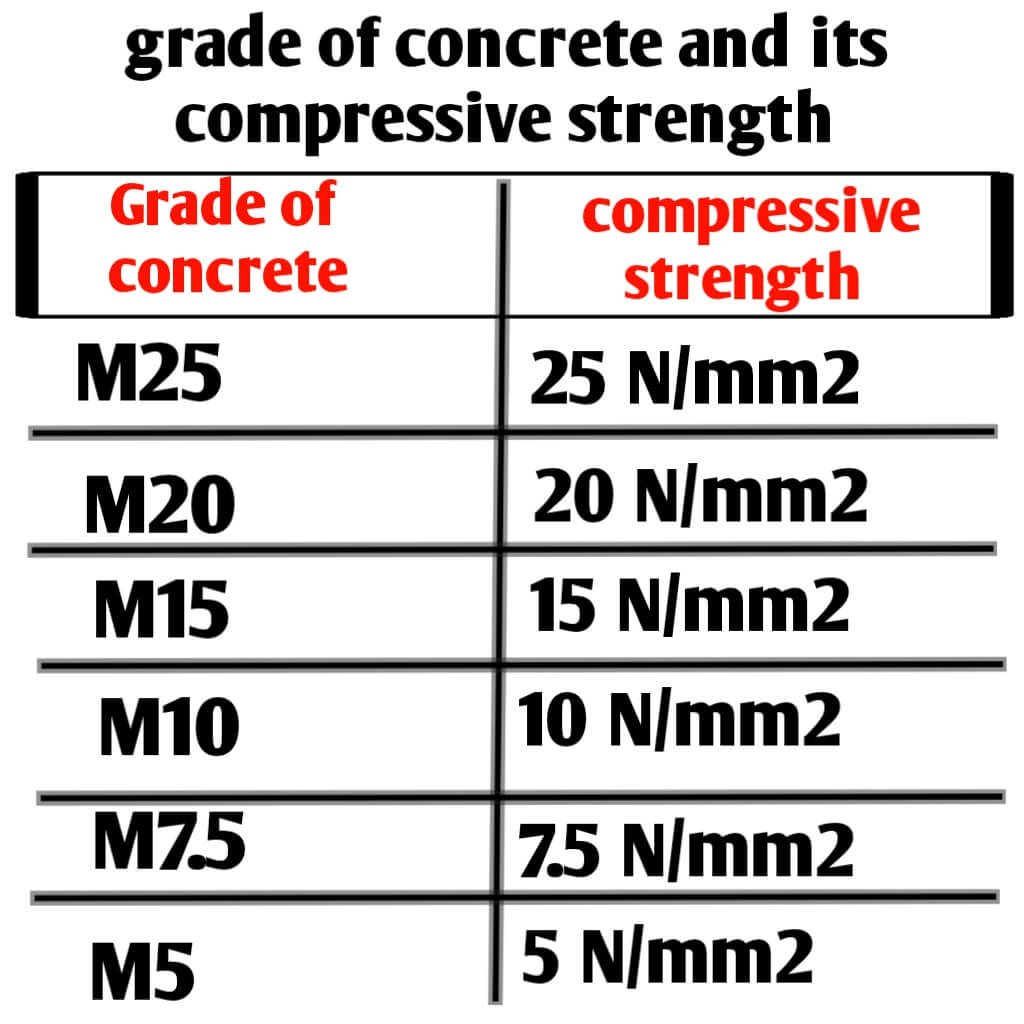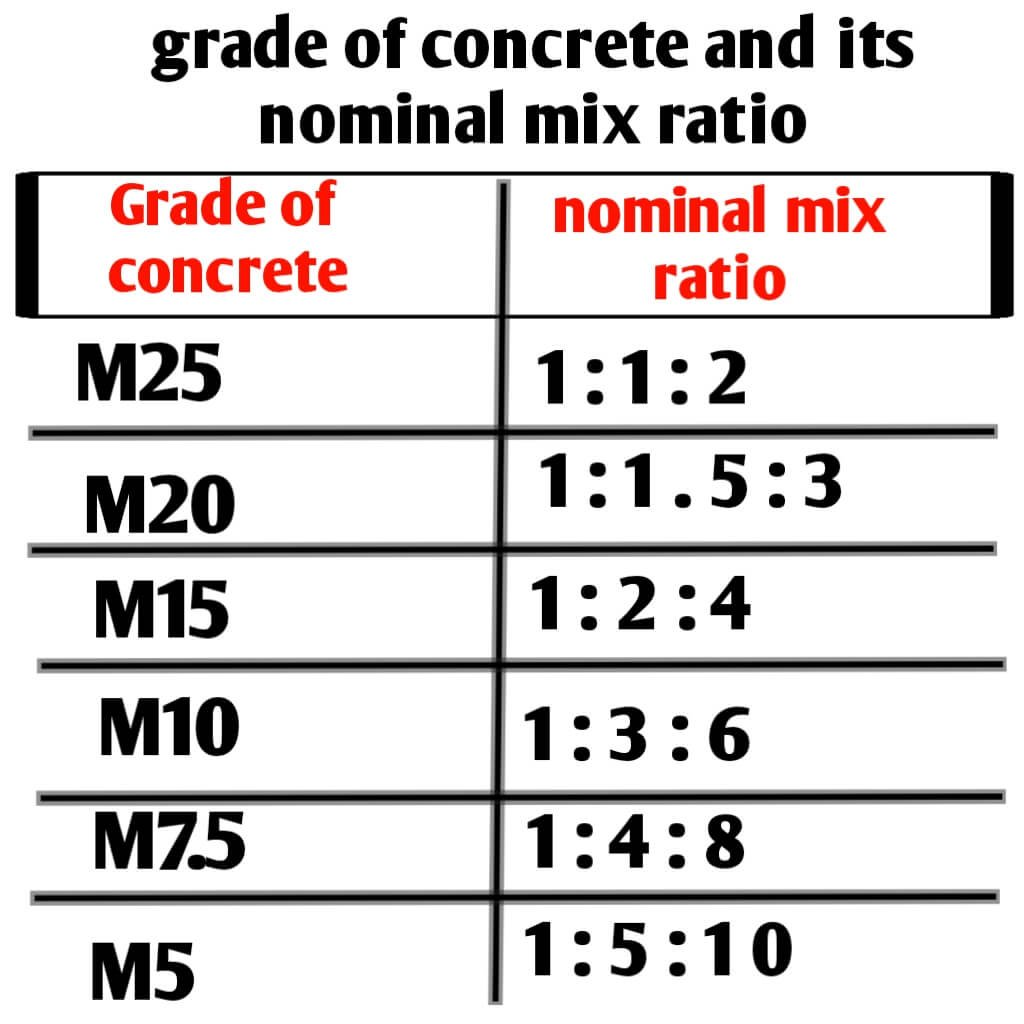# Concrete mix ratio Table | concrete grade types

concrete mix ratio Table and concrete grade types, hi guys in this article we know about different types of concrete grade and their uses, we know concrete mix ratio for roof slab, column, beam, Foundation and floor. And discuss about concrete mix ratio m5,m7.5,m10,m15,m20 and m25.

We know that concrete each mixture of cement sand and aggregate in fixed proportion and concrete is used for several work in Civil Engineering construction of high rise and low rise building, over bridge, Bridge, dams, retaining walls and different types of piles.

Concrete mix ratio is used as PCC (plain cement concrete) in Roadways Pathwas formation and for flooring of house and also concrete mix ratio is used as RCC for the formation of roof slab, Foundation, footing, beam and column.Types of concrete grade and their ratio

We discuss about concrete grade types according to their use, there are two types of concrete grade given following:-

1) PCC (plain cement concrete)

2) RCC (reinforce cement concrete)

### PCC ( plain cement concrete)

1) PCC :- it is plain cement concrete which has mixture of cement sand and aggregate which is used for mainly flooring and formation of different types of Pathways. PCC have do not use of Steel bar, concrete without use of Steel bar is used as and known as PCC

### RCC (Reinforced cement concrete)

2) RCC :- it is reinforced cement concrete mixture of cement sand and aggregate with steel bar and used for formation of different types of civil construction works like beam, slab, footing, column , bridge, dams and piles. Concrete mix with steel bar known as RCC.

### concrete grade types according to their mix

1) nominal mix concrete

2) design mix concrete

1) nominal mix concrete :- it is simple type of concrete mix used in domestic and formation of low rise building, M5 M10 m15 M20 and M25 is used in domestic purpose for different type of residential house and formation of bass for foundation. ratio of in this grade of concrete has fixed ratio of sand cement and aggregate.

That’s why it is known as nominal mix concrete like mix ratio in M20 grade of concrete is about 1:1.5:3 in which one part is cement 1.5 part is sand and 3 part is aggregate.

2) design mix concrete:- it is standard type of concrete mix in which mix ratio of cement sand and aggregate is decided by expert engineer according to require strength of concrete and requirement of concrete strength for high rise building over bridge dams formation of piles.

mainly mix ratio is calculated and decided by engineer according to requirement of strength of civil work construction. So grade above m25 like M30 m35 m40 m50 and so on has no fixed mix ratio of concrete that’s why it is called design mix concrete.

● Table for concrete grade types according to their concrete mix ratio:-Table for concrete grade types according to their concrete mix ratio

### Concrete grade types according to their strength

■1) ordinary concrete grade :– it is simple type of concrete grade mainly used for flooring of surface and formation of flat base for footing and filling , concrete grade like M5 M10 m15 and M20 is ordinary concrete grade, in which M stand for mix and there numerical figure 5 10 15 and 20 stand for comprehensive strength of concrete in 28 days of curing time.

M5 M10 and m15 concrete is not used for RCC work it is only used for PCC work and nominal mix concrete M20 is only ordinary concrete grade which is used in both PCC and RCC work construction of low rise building.

2) standard grade of concrete:- it is standard grade of concrete used for high rise building construction ,formation of over bridge ,dams ,Bridge and different types of foundation piles, and Railway sleeper. M25 M30 m35 m40 and m45 is known as standard grade of concrete used for RCC work.

Only m25 grade of concrete has nominal mix ratio of cement sand and aggregate that is 1:1:2 and above m25 grade of concrete has no fix ratio of cement sand and aggregate it is calculated and decided by export engineer according to requirement of strength of construction work.

◆3) high strength grade of concrete :- it has higher strength than ordinary and standard grade of concrete, m50,m55 m60 m70 is high strength grade of concrete, it is commercially used for construction of high rise building according to requirement of strength and their strength and mix ratio of cement sand and aggregate is also calculated and decided by expert engineer.

● Table for compressive strength of different concrete grade types:-different grade of concrete and their compressive strength in N/mm2.

## Concrete mix ratio Table

There are different grade of concrete  and their mix ratio is discuss and concrete mix ratio table is given below:-

● Table for concrete mix ratio:-Concrete mix ratio Table

### Concrete mix ratio M5 (1:5:10)

Concrete mix ratio M5 (1:5:10) :- it is ordinary grade of concrete basically it is not used for structural work and concrete mix ratio (cement sand and aggregate) in M5 grade of concrete is 1:5:10, in which one part is cement 5 Part is sand and 10 part is aggregate.

And comprehensive strength of M5 grade of concrete is 5 mega pascal which gain in 28 days of curing time

### Concrete mix ratio M7.5 (1:4:8)

Concrete mix ratio M7.5 (1:4:8) :- it is ordinary grade of concrete and also not used for structural work it is mainly used for flooring, PCC and Flat base surface for footing and it has concrete mix ratio (cement, sand and aggregate) for M7.5 is 1:4:8, in which one part is cement 4 part is sand and 8 part is aggregate,

And comprehensive strength of M7.5 grade of concrete is 7.5 mega pascal which gain in 28 days of curing time

### Concrete mix ratio M10 (1:3:6)

Concrete mix ratio M10 (1:3:6) :- it is ordinary grade of concrete and also not used for structural work it is mainly used for flooring, PCC and Flat base surface for footing and it has concrete mix ratio (cement, sand and aggregate) for M10 is 1:3:6, in which one part is cement 3 part is sand and 6 part is aggregate,

And comprehensive strength of M10 grade of concrete is 10 mega pascal which gain in 28 days of curing time

### Concrete mix ratio M15 (1:2:4)

Concrete mix ratio M15 (1:2:4) :- it is also ordinary grade of concrete used for PCC work and flooring of surface not used for RCC work, nominal mix ratio in m15 grade of concrete is about 1:2:4 in which one part is cement 2 part is sand and 4 part is aggregate.

And comprehensive strength of M15 grade of concrete is 15 mega pascal which gain in in 28 days of curing time

### Concrete mix ratio M20 (1:1.5:3)Types of concrete grade and their ratio

Concrete mix ratio M20:- it is only ordinary grade of concrete which is used in RCC work for domestic uses for construction of low rise building and concrete mix ratio for m20 is 1:1.5:3, in which one part is cement, 1.5 part is sand and 3 part is aggregate.

M20 grade of concrete used for structural work for footing, beam, column and slab. And comprehensive strength of M20 grade of concrete is 20 mega pascal which gain in in 28 days of curing time

You should also visits:-

1)what is concrete and its types and properties

2) concrete quantity calculation for staircase and its formula

3) how to calculate weight of mild steel plate and derive its formula

4) calculate quantity of cement Sand for brickwork of 10m3

5) cement calculation in tile work of hundred square foot area

6) weight calculation of Steel bar and its formula

7) what is admixture of concrete and its types and its properties

### Concrete mix ratio M25 (1:1:2)

Concrete mix ratio M25 :- it is Standard grade of concrete mainly used for RCC work in domestic uses for the construction of beam, slab, footing and column , and concrete mix ratio for m25 is 1:1:2, in which one part is cement 1 part is sand and 2 part is aggregate.

And comprehensive strength of M25 grade of concrete is 25 mega pascal which gain in in 28 days of curing time.

### Concrete mix ratio for roof slab

As we know that roof slab is RCC structure so we can use minimum grade of M20 grade and concrete mix ratio 1:1.5:3 for roof slab & maximum grade of concrete m25, M30 and much higher are used as per design for roof slab.

1:1.5:3 (m20) is concrete mix ratio used for roof slab.

### Concrete mix ratio for beams

As we know that Beam is RCC structure so we can use minimum grade of M20 grade and concrete mix ratio 1:1.5:3 for beams & maximum grade of concrete m25, M30 and much higher are used as per design for beam.

1:1.5:3 (m20) is concrete mix ratio used for beam.

### Concrete mix ratio for Foundation

As we know that Foundation footing is RCC structure so we can use minimum grade of M20 grade and concrete mix ratio 1:1.5:3 for Foundation & maximum grade of concrete m25, M30 and much higher are used as per design for Foundation footing.

1:1.5:3 (m20) is concrete mix ratio used for Foundation footing.

### Concrete mix ratio for column

As we know that column is RCC structure so we can use minimum grade of M20 grade and concrete mix ratio 1:1.5:3 for column & maximum grade of concrete m25, M30 and much higher are used as per design for column.

1:1.5:3 (m20) is concrete mix ratio used for column.

### Concrete mix ratio for floor

As we know that floor is non RCC structure so we can use minimum grade of M7.5 grade and concrete mix ratio 1:4:8 for floor & grade of concrete m10 & M15 are also used for floor.

1:4:8 (m7.5), 1:3:6 (m10) and 1:2:5 (m15) is concrete mix ratio used for floor, PCC & none RCC structure.